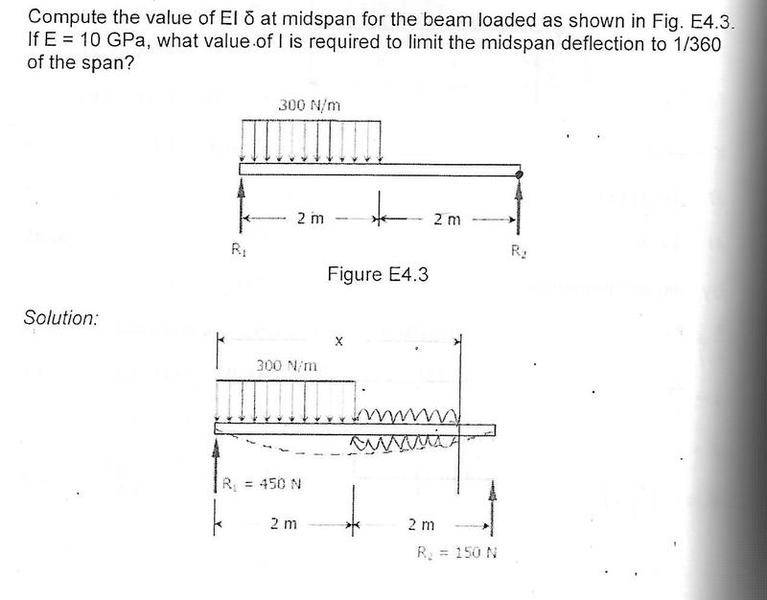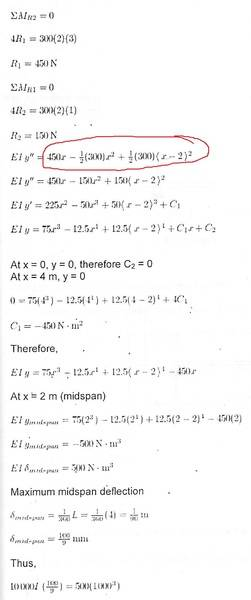# Moment in beam

## Homework Statement

refer to the circled part , i dont understand the author's working .## The Attempt at a Solution

I think it should be 450x - 150(4-x) -30092)(x-1) , correct me if i'm wrong . (taking moment about x ) ....

is the author wrong , coz the distributed load start from R1 to 2m from R1 ...

SteamKing
Staff Emeritus
Homework Helper
is the author wrong , coz the distributed load start from R1 to 2m from R1 ...
No, the author is not wrong.

The bending moment function EIy" is carefully constructed to show that the distributed load starts at x = 0 and runs to x = 2, after which an equal and opposite distributed load is applied to the beam at x > 2, so that the net distributed load is zero.

•No, the author is not wrong.

The bending moment function EIy" is carefully constructed to show that the distributed load starts at x = 0 and runs to x = 2, after which an equal and opposite distributed load is applied to the beam at x > 2, so that the net distributed load is zero.
why ? i still dont understand , i can only understand the 450x , why should be -0.5(300)(x^2) +0.5(300)[(x-2)^2 ]

No, the author is not wrong.

The bending moment function EIy" is carefully constructed to show that the distributed load starts at x = 0 and runs to x = 2, after which an equal and opposite distributed load is applied to the beam at x > 2, so that the net distributed load is zero.
why shouldnt it be 450x - 150(4-x) -300(0.5)(2)(x-1) ??

SteamKing
Staff Emeritus
Homework Helper
why shouldnt it be 450x - 150(4-x) -300(0.5)(2)(x-1) ??
I'm not going to speculate on that.

Look, the author has a method of analysis of this beam which he is trying to teach. If you want to develop your own methods, write your own textbook.

•I'm not going to speculate on that.

Look, the author has a method of analysis of this beam which he is trying to teach. If you want to develop your own methods, write your own textbook.
i dont understand why it is -0.5(300)(x^2) +0.5(300)[(x-2)^2 ] , can you explain ?

No, the author is not wrong.

The bending moment function EIy" is carefully constructed to show that the distributed load starts at x = 0 and runs to x = 2, after which an equal and opposite distributed load is applied to the beam at x > 2, so that the net distributed load is zero.
why it's 0.5(300)(x^2) ? the 300N/m only 'defined ' from R1 to 2m from R1, am i right ? why shouldnt it be 0.5(300)(2)(x-2) instead? as you said , after 2m , net distributed load is zero

I'm not going to speculate on that.

Look, the author has a method of analysis of this beam which he is trying to teach. If you want to develop your own methods, write your own textbook.
the location of 300n/m is at R1 and extend to 2m from R1, so ,the EIy" should be = 450x-300(2)(x-1) ???
why there's -0.5(300)(x^2) +0.5(300)( (x-2)^2 )????
I'm confused

Or do you mean the 300(X) force still exist at X >2?

Last edited:
bump